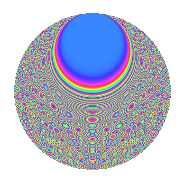# Properties

 Label 1575.2.ckLevel 1575 Weight 2 Character orbit ck Rep. character $$\chi_{1575}(218,\cdot)$$ Character field $$\Q(\zeta_{12})$$ Dimension 432 Sturm bound 480

# Related objects

## Defining parameters

 Level: $$N$$ = $$1575 = 3^{2} \cdot 5^{2} \cdot 7$$ Weight: $$k$$ = $$2$$ Character orbit: $$[\chi]$$ = 1575.ck (of order $$12$$ and degree $$4$$) Character conductor: $$\operatorname{cond}(\chi)$$ = $$45$$ Character field: $$\Q(\zeta_{12})$$ Sturm bound: $$480$$

## Dimensions

The following table gives the dimensions of various subspaces of $$M_{2}(1575, [\chi])$$.

Total New Old
Modular forms 1008 432 576
Cusp forms 912 432 480
Eisenstein series 96 0 96

## Trace form

 $$432q - 4q^{3} + O(q^{10})$$ $$432q - 4q^{3} + 24q^{11} + 16q^{12} + 216q^{16} + 64q^{18} + 8q^{21} + 24q^{23} + 32q^{27} + 60q^{32} + 16q^{33} + 32q^{36} + 24q^{37} - 72q^{38} - 96q^{41} + 40q^{42} + 96q^{46} - 12q^{47} - 104q^{48} - 88q^{51} + 4q^{57} - 8q^{63} + 40q^{66} + 12q^{67} + 64q^{72} + 48q^{76} - 72q^{78} - 184q^{81} + 96q^{82} - 120q^{83} - 72q^{86} - 116q^{87} + 48q^{88} - 48q^{91} - 156q^{92} + 44q^{93} + 192q^{96} + 60q^{97} + O(q^{100})$$

## Decomposition of $$S_{2}^{\mathrm{new}}(1575, [\chi])$$ into newform subspaces

The newforms in this space have not yet been added to the LMFDB.

## Decomposition of $$S_{2}^{\mathrm{old}}(1575, [\chi])$$ into lower level spaces

$$S_{2}^{\mathrm{old}}(1575, [\chi]) \cong$$ $$S_{2}^{\mathrm{new}}(45, [\chi])$$$$^{\oplus 4}$$$$\oplus$$$$S_{2}^{\mathrm{new}}(225, [\chi])$$$$^{\oplus 2}$$$$\oplus$$$$S_{2}^{\mathrm{new}}(315, [\chi])$$$$^{\oplus 2}$$

## Hecke Characteristic Polynomials

There are no characteristic polynomials of Hecke operators in the database